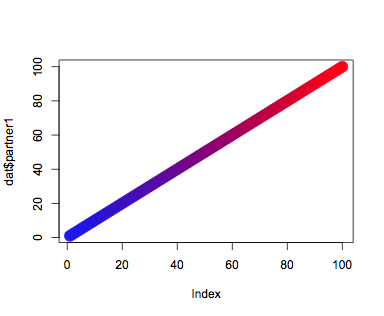Biostar Beta. Not for public use.
plotting interactions in R with two data sets
1
Entering edit mode
6.3 years ago
frymor • 10
European Union

Hi all,

I have a data set of two postions on the genome with a third value for number of interactions. I would like to plot this data set so I can see how many interactions are on each position.

the data set looks like that (this is only a subset of the complete, very long list):

```partner1 partner2 Interactions 1 10001 11 1 15001 1 1 20001 1 1 25001 4 1 30001 8 5001 20001 1 5001 40001 3 5001 45001 15 5001 50001 1 10001 15001 3 10001 20001 3 10001 25001 6 10001 30001 12 15001 70001 2 15001 90001 6 15001 95001 5 15001 100001 1 20001 4195001 30 20001 4200001 62 20001 4205001 81 20001 4210001 3 25001 30001 5 25001 40001 22 25001 45001 13 4200001 4210001 318 4200001 4215001 2 4205001 4210001 308 4205001 4215001 2 4210001 4215001 1```

i would like to have the column 'partner1' on the x-axis, the column 'partner2' on the y-axis and the number of interactions (3rd column) in the plot with the option to have there either a point, the number itself of a colored gradient like in the heatmaps.

Does anyone know of an R package for creating such plots, or for that matter, any other way of doing it?

thanks

Assa

1
Entering edit mode

i think the best way to represent this sort of data would be with a heatmap. is there a directionality between partner one and partner 2? e.g. the values "1 5000 8" are different from "5000 1 8" in your table

0
Entering edit mode

yes there is a difference. The information on the two partner columns are genomic positions. So it make a difference whether the first or the second partner is on a specific position. Doesn't it?

How would you put the data into a heatmap?

4
Entering edit mode
17 months ago
Irsan ♦ 6.9k
Amsterdam

There are many possibilities, one of them is using ggplot2 (R-library)

`library(ggplot2)`

`ggplot(data) + geom_tile(aes(x=factor(partner1),y=factor(partner2),fill=Interactions))`0
Entering edit mode

I have tried with ggplot.

```require(ggplot2) pl1 <- ggplot(subset, aes(y = factor(partner1), x = factor(partner2))) + geom_tile(aes(fill = Interactions)) + scale_fill_continuous(low = "blue", high = "green") + scale_size(range = c(1, 200))```

With the small subset I get a similar plot to the one you posted. But with the complete data set I get a different picture.

Is there a simple explanation for that? Does the order of the columns of the two partner columns make a difference?

thanks

1
Entering edit mode

`data\$partner1 <- factor(data\$partner1, levels=sort(unique(data\$partner1)))`

(and also for partner2) then plot without the factor() part

0
Entering edit mode

That still didn't change anything. I still get the plot on only half of the window. I can't figure why, as I have for both columns the same amount of factors (842 vs. 843).

0
Entering edit mode

is it possible to make the legend a bit more comprehensive? I won't to have more than just 5 different categories. I need a much bigger separation - something like 20 or 25 different color points.

4
Entering edit mode
15 months ago
WCIP | Glasgow | UK

## Dummy data

`dat<- data.frame(partner1= 1:100, partner1= 1:100, Interactions= 1:100)`

```ncols<- length(unique(dat\$Interactions)) cols<- data.frame( colour= colorRampPalette(c("blue", "red"))(ncols), Interactions= sort(unique(dat\$Interactions)), stringsAsFactors= FALSE)```

`dat<- merge(dat, cols)`

`## Unocmment to Make colour transparent, it might look better

# dat\$colour<- paste(dat\$colour, trasp, sep= '')`

```## Plot symbol plot(x= dat\$partner1, y= dat\$partner2, pch= 19, col= dat\$colour, cex= 2)```

```## As text plot(x= dat\$partner1, y= dat\$partner2, type= 'n') text(x= dat\$partner1, y= dat\$partner2, labels= dat\$Interactions, col= dat\$colour, cex= 0.5)```0
Entering edit mode

Thanks I will give it a try...

1
Entering edit mode
4.3 years ago
t.candelli • 60
France

i'm going to use the "pheatmap" package to draw a heatmap of your data. with the code below i generate a matrix from your dataframe so that it can be used as an argument for pheatmap.

`library(pheatmap)`

```names<-unique(c(data[,1], data[,2])) mat<-matrix(data=0, nrow=length(names), ncol=length(names)) rownames(mat)<-sort(names) colnames(mat)<-sort(names)```

`for (i in 1:nrow(data))
{
partner1 <- as.character(data[i,1])
partner2 <- as.character(data[i,2])
interactions <- data[i,3]

mat[partner1, partner2] <- interactions
}`

`pheatmap(mat, cluster_cols=F, cluster_rows=F)`

0
Entering edit mode
21 months ago
Marseille, France

A good solution to such a problem is to draw a network representation where:

- partners are nodes

- column 3 is the thickness of the link

THE SOFT for that is Cytoscape